# Coax Loss due to Loss Tangent

On this page we will derive the equation for loss due to loss tangent in coax. Boring stuff for most people, but I makes us feel good when algebra works out so well! Note that if you are dealing with air coax, loss tangent is zero, and you don't have to deal with this term!

First we start out with the inductance/length and capacitance per length formulas for coax. Note the "prime" notation, we'll try to consistently use this when quantities are normalized per unit length. For everything on this page, assume that the length units are meters to avoid confusion.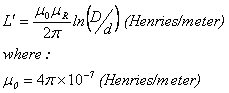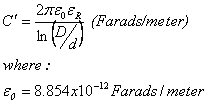Plugging the L' and C' equations into the definition of characteristic impedance  (Z0) from Telegrapher's Equations we get: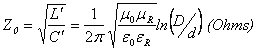If we assume that μR=1, we can come up with the familiar equation for Z0 (which is here for reference, we don't need to simplify this formula for the subject at hand):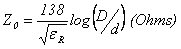Next we go back to the capacitance/length equation and calculate capacitive susceptance per unit length: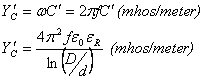The loss tangent introduces a "real" conductance which is calculated from the susceptance by multiplying by loss tangent.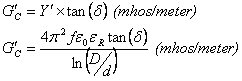Now it's time to look at the generic formula for αD: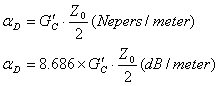We plug in Gprime and Z0 into this and get attenuation in Nepers/meter: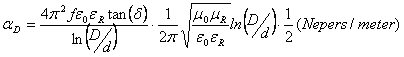If we substitute f=c/λ and simplify, then: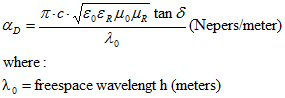Until March 2017, the above equation was missing the "c" in the numerator, we apologize for any problems that might have caused. Thanks to Stefan for correcting it!

Now the final step is to assume μR=1, and note that one dB=8.686 Nepers, and multiply all of the constants together to get the "classic" formula: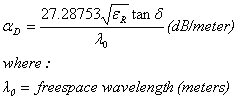In the above formula you can use any units you like for wavelength, to get the loss/length in those units.

Here we'll change the formula so you can plug in frequency (in GHz), we find that more convenient: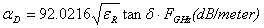So loss due to loss tangent is exactly proportional to frequency, while metal losses are proportional to SQRT frequency. The implication here is that at higher and higher frequency, eventually loss tangent will start to dominate the total loss of the coax line.

Author : Unknown Editor